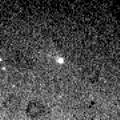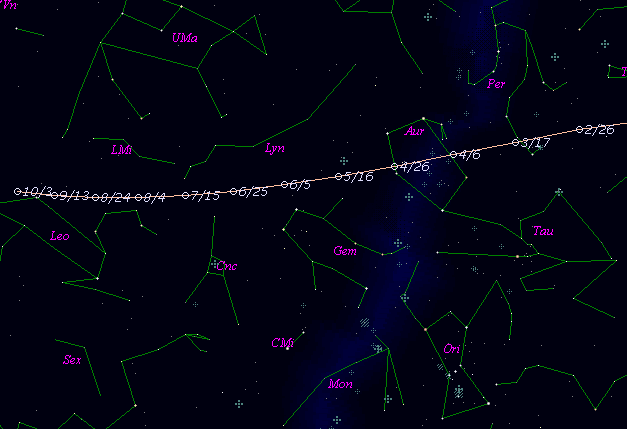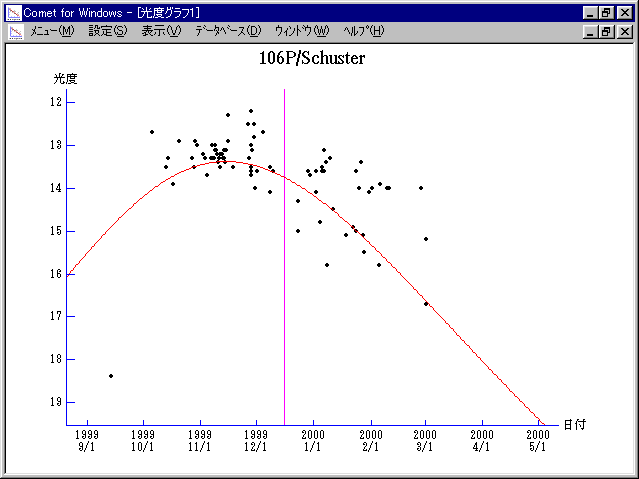# \$B%7%e%9%?!

106P/Schuster (1999)###\$B###\$B50F;MWAG(B

```   The following improved orbital elements by Kenji Muraoka, are
from 141 observations  1977 to 2000, including 9 Planets, Moon
and 5 minor planets perturbations and non-gravitational effect
of style II.   The mean residual is +/- 0.75 arc seconds.

Epoch  =  1999 Dec.  8.0  TT       JDT = 2451520.5
T  =  1999 Dec. 16.22615       +/- 0.00046 (m.e.) TT
Peri. =  355.85857                +/- 0.00031
Node  =   50.58924                +/- 0.00005   (2000.0)
Incl. =   20.13872                +/- 0.00002
q  =    1.5497182              +/- 0.0000023 AU
e  =    0.5879217              +/- 0.0000006
a  =    3.7607372              +/- 0.0000020 AU
n  =    0.13514333             +/- 0.00000011
P  =    7.293                  +/- 0.0000059  years
A1  =   +0.096                  +/- 0.022
A2  =   +0.00716                +/- 0.00038
```

###\$B@1?^(B###\$B8wEYJQ2=(B

```        m1 = 9 + 5 log\$B&\$(B + 25 log r(t + 15)
```##### \$B50F;MWAG\$OB<2,7r<#;a\$N7W;;\$K\$h\$k\$b\$N\$G\$9!#(B \$B@1?^\$O(B StellaNavigator Ver.2.0 for Windows (\$B%"%9%H%m%"!<%D(B \$BJTCx(B / \$B%"%9%-!<=PHG6I4)(B) \$B\$G:n@.\$7\$?\$b\$N\$G\$9!#(B \$B8wEY%0%i%U\$O(BComet for Windows\$B\$G:n@.\$7\$?\$b\$N\$G\$9!#(B